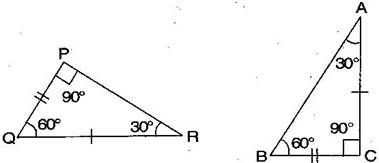# Congruence of triangles worksheet for class 7## myCBSEguide App

CBSE, NCERT, JEE Main, NEET-UG, NDA, Exam Papers, Question Bank, NCERT Solutions, Exemplars, Revision Notes, Free Videos, MCQ Tests & more.

## Congruence of triangles worksheet for class 7 Important Topics

• The idea of congruence in geometry
• Congruence of plane figures, line segments, angles
• Criteria for congruence of triangles
• Congruence among right-angled triangles## Some important Facts about Congruence of triangles worksheet for class 7

1. ASA Congruence of two triangles: Under a given correspondence, two triangles are congruent if two angles and the side included between them in one of the triangles are equal to the corresponding angles and the side included between them of the other triangle.
2. RHS Congruence of two right-angled triangles: Under a given correspondence, two right-angled triangles are congruent if the hypotenuse and a leg of one of the triangles are equal to the hypotenuse and the corresponding leg of the other triangle.
3. There is no such thing as AAA Congruence of two triangles: Two triangles with equal corresponding angles need not be congruent. In such a correspondence, one of them can be an enlarged copy of the other. (They would be congruent only if they are exact copies of one another).

## NCERT class 7Mathematics Solved Worksheets

• Chapter 1 – Integers
• Chapter 2 – Fractions and Decimals
• Chapter 3 – Data Handling
• Chapter 4 – Simple Equations
• Chapter 5 – Lines and Angles
• Chapter 6 – Practical Geometry
• Chapter 7 – The Triangle and its Properties
• Chapter 8 – Congruence of triangles
• Chapter 9 – Comparing Quantities
• Chapter 10 – Rational numbers
• Chapter 11 – Perimeter and Area
• Chapter 12 – Algebraic Expressions
• Chapter 13 – Exponents and Powers
• Chapter 14 – Symmetry
• Chapter 15 – Visualizing Solid Shapes

## CONGRUENCE AMONG RIGHT-ANGLED TRIANGLES

Congruence in the case of two right triangles deserves special attention. In such triangles, obviously, the right angles are equal. So, the congruence criterion becomes easy. Can you draw ∆ABC (shown in Fig 7.29) with ∠B = 90°, if

1. only BC is known?
2. only ∠C is known?
3. ∠A and ∠C are known?
4. AB and BC are known?
5. AC and one of AB or BC are known?

Try these with rough sketches. You will find that (iv) and (v) help you to draw the triangle. But case (iv) is simply the SAS condition. Case (v) is something new. This leads to the following criterion:

To download Printable worksheets for class 7 Mathematics and Science; do check myCBSEguide app or website. myCBSEguide provides sample papers with solution, test papers for chapter-wise practice, NCERT solutions, NCERT Exemplar solutions, quick revision notes for ready reference, CBSE guess papers and CBSE important question papers. Sample Paper all are made available through the best app for CBSE students and myCBSEguide website.Test Generator

Create question papers online with solution using our databank of 5,00,000+ questions and download as PDF with your own name & logo in minutes.